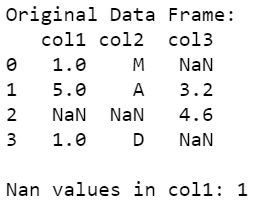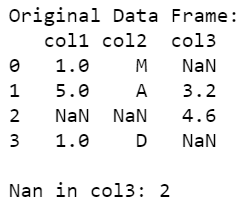Related Articles

# How to Count the NaN Occurrences in a Column in Pandas Dataframe?

• Last Updated : 11 Dec, 2020

The data frame is divided into cells, which can store a value belonging to some data structure as well as it may contain missing or NA values. The pandas package contains various in-built functions, to check if the value in the cell of a data frame is either NA or not, and also to perform aggregations over these NA values.

Method #1: Using In-built methods isna() and sum() on the dataframe.

The isna() function is used to detect missing/none values and return a boolean array of length equal to the data frame element over which it is applied and the sum() method is used to calculate a total of these missing values.

## Python3

 `# importing necessary packages``import` `pandas as pd``import` `numpy as np`` ` `# creating data``data ``=` `[[``1``, ``"M"``, np.nan], [``5``, ``"A"``, ``3.2``], [``    ``np.nan, np.nan, ``4.6``], [``1``, ``"D"``, np.nan]]`` ` `# converting data to data frame``data_frame ``=` `pd.DataFrame(data, ``                          ``columns``=``[``"col1"``, ``"col2"``, ``"col3"``])`` ` `# printing original data frame``print``(``"\nOriginal Data Frame:"``)``print``(data_frame)`` ` `# counting NaN values of col1``cnt ``=` `data_frame[``"col1"``].isna().``sum``()`` ` `# printing count of NaN values``print``(``"\nNan values in col1:"``, cnt)`

Output:Method #2: Using the length of the dataframe

The count of the values contained in any particular column of the data frame is subtracted from the length of dataframe, that is the number of rows in the data frame. The count() method gives us the total number of NaN values in a specified column and the length(dataframe) gives us the length of the data frame, that is the total number of rows in the frame.

## Python3

 `# importing necessary packages``import` `pandas as pd``import` `numpy as np`` ` `# creating data``data ``=` `[[``1``, ``"M"``, np.nan], [``5``, ``"A"``, ``3.2``],``        ``[np.nan, np.nan, ``4.6``], [``1``, ``"D"``, np.nan]]`` ` `# converting data to data frame``data_frame ``=` `pd.DataFrame(data, columns``=``[``"col1"``, ``"col2"``, ``"col3"``])`` ` `# printing original data frame``print``(``"\nOriginal Data Frame:"``)``print``(data_frame)`` ` `# counting NaN values of col1``length ``=` `len``(data_frame)``count_in_col3 ``=` `data_frame[``'col3'``].count()``cnt ``=` `length ``-` `count_in_col3`` ` `# printing count of NaN values``print``(``"\nNan in col3:"``, cnt)`

Output:Attention geek! Strengthen your foundations with the Python Programming Foundation Course and learn the basics.

To begin with, your interview preparations Enhance your Data Structures concepts with the Python DS Course. And to begin with your Machine Learning Journey, join the Machine Learning – Basic Level Course

My Personal Notes arrow_drop_up